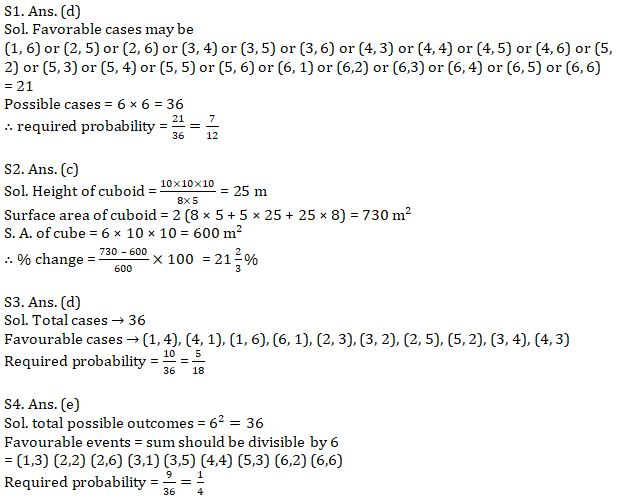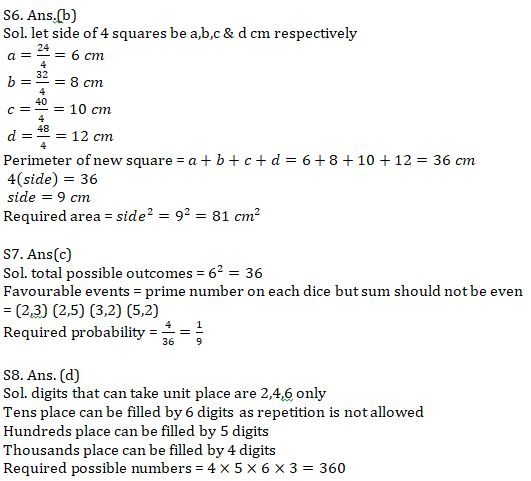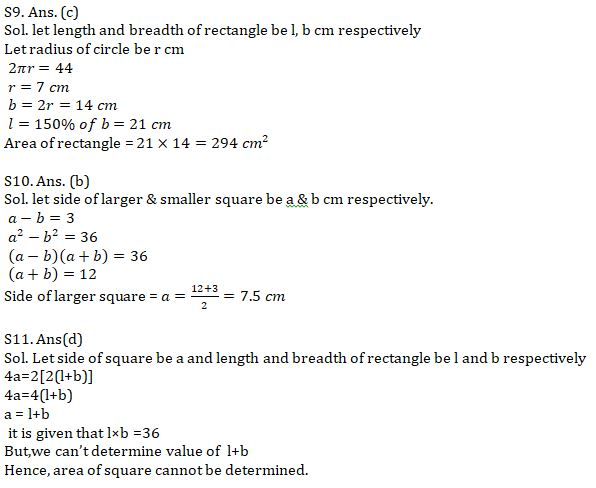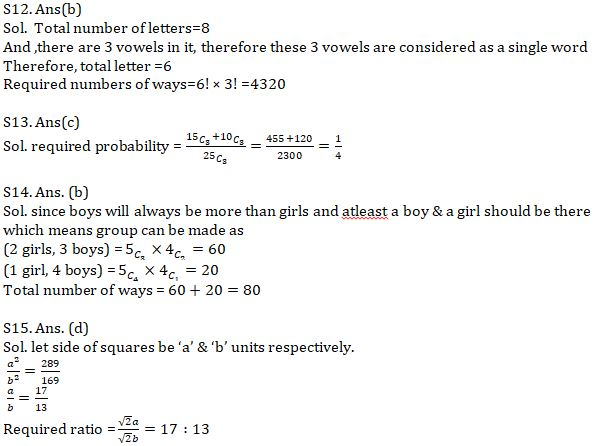Latest Banking jobs   »   Quantitative Aptitude Quiz For IBPS Clerk...

# Quantitative Aptitude Quiz For IBPS Clerk Prelims 2021- 4th August

Q1. Two dice are thrown simultaneously. Find the probability of getting a sum of two numbers greater than 6.
(a) 3/4
(b) 23/36
(c) 5/12
(d) 7/12
(e) 11/36

Q2. An ice cube of side 10 m is melted and re-casted into a cuboid having length and breadth are 8m and 5 m respectively. Find the percentage of difference between total surface area of cube and cuboid.
(a) 25(2/3) %
(b) 23(2/3) %
(c) 21(2/3) %
(d) 26 %
(e) None of these

Q3. A man have two dice, if he rolled both dice, then find the probability that sum of digits will be 5 or 7 ?
(a) 1/18
(b) 1/12
(c) 5/9
(d) 5/18
(e) 7/18

Q4. Two dice are thrown simultaneously. What is the probability of getting sum of numbers obtained on two dice divisible by 4?
(a) 4/9
(b) 1/18
(c) 1/9
(d) 1/6
(e) 1/4

Q5. How many words can be formed with the letter of the word UNETHICAL such that all vowels come together?
(a) 720
(b) 10!
(c) 5040
(d) 17280
(e) None of these

Q6. The perimeter of 4 squares is 24 cm, 32 cm, 40 cm, 48 cm respectively. What will be the area of the square having perimeter equal to sum of edges of 4 squares? (in sq.cm.)
(a) 64
(b) 81
(c) 100
(d) 121
(e) 144

Q7. Two dice are thrown simultaneously. What is the probability of getting prime numbers on both and sum of numbers on both dice is not divisible by 2?
(a) 1/12
(b) 1/18
(c) 1/9
(d) 1/6
(e) None of these

Q8. How many 4 digit numbers can be made from digits 1,2,3,4,5,6,7 such that each no. is divisible by 2 and no digit repeats?
(a) 720
(b) 120
(c) 480
(d) 360
(e) None of these

Q9. Breadth of a rectangle is equal to diameter of circle of which circumference is 44 cm. if length of rectangle is 50% more than its breadth. Find area (in sq. cm.) of rectangle.
(a) 292
(b) 296
(c) 294
(d) 298
(e) None of these

Q10. Two squares are drawn on a same base but of different edge length. If difference of their area is 36 sq.cm. find the edge length of larger square if difference of their edge length is 3 cm.
(a) 5.5 cm
(b) 7.5 cm
(c) 6.5 cm
(d) 4.5 cm
(e) 6 cm
Q11. The perimeter of a square is double than the perimeter of a rectangle. The area of the rectangle is 36 sq.cm. what is the area of square?
(a) 72 sq.cm
(b) 56 sq.cm
(c) 64 sq.cm
(d) can’t be determined
(e) 108 sq.cm

Q12. In how many different ways can the letter of word ‘champion’ be arranged so that all the vowels come together?
(a)4820
(b) 4320
(c) 4640
(d) 5280
(e)None of these
Q13. A storekeeper has 2 types of shirts i.e. 15 yellow and 10 blue. If he sold 3 shirts,
what is the probability that these are either all yellow or all blue?
(a)1/2
(b) 3/10
(c) 1/4
(d) 28/115
(e) 3/25

Q14. How many ways are there to form a committee of 5 people from 5 boys and 4 girls such that number of boys will always be more than number of girls and atleast a boy & a girl should be there in the committee?
(a) 60
(b) 80
(c) 100
(d) 85
(e) None of these

Q15. The ratio of areas of two squares is 289 : 169. Find the ratio of their diagonals.
(a) 19 : 15
(b) 15 : 13
(c) 17 : 15
(d) 17 : 13
(e) 13 : 11

Solutions#### Congratulations!Union Budget 2023-24: Free PDF